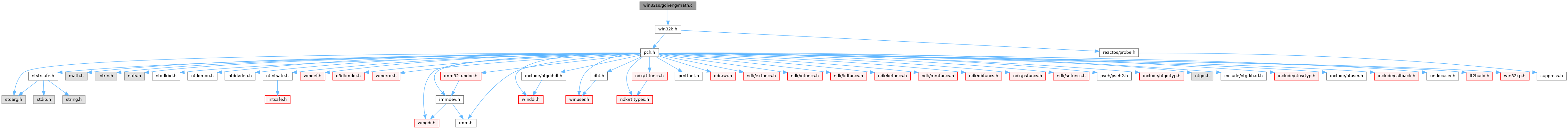ReactOS  0.4.15-dev-3723-g8d70159
math.c File Reference
#include <win32k.h>
Include dependency graph for math.c:Go to the source code of this file.

Functions

INT APIENTRY EngMulDiv (_In_ INT iMultiplicand, _In_ INT iMultiplier, _In_ INT iDivisor)

◆ EngMulDiv()

 INT APIENTRY EngMulDiv ( _In_ INT iMultiplicand, _In_ INT iMultiplier, _In_ INT iDivisor )

Definition at line 26 of file math.c.

30 {
31  INT64 i64Multiplied, i64Result;
32
33  /* Check for divide by zero */
34  if (iDivisor == 0)
35  {
36  /* Quick sign check and return "infinite" */
37  return ((iMultiplicand ^ iMultiplier) < 0) ? INT_MIN : INT_MAX;
38  }
39
40  /* We want to deal with a positive divisor to simplify the logic. */
41  if (iDivisor < 0)
42  {
43  iMultiplicand = -iMultiplicand;
44  iDivisor = -iDivisor;
45  }
46
47  /* Do the multiplication */
48  i64Multiplied = Int32x32To64(iMultiplicand, iMultiplier);
49
50  /* If the result is positive, we add to round, else we subtract to round. */
51  if (i64Multiplied >= 0)
52  {
53  i64Multiplied += (iDivisor / 2);
54  }
55  else
56  {
57  i64Multiplied -= (iDivisor / 2);
58  }
59
60  /* Now do the divide */
61  i64Result = i64Multiplied / iDivisor;
62
63  /* Check for positive overflow */
64  if (i64Result > INT_MAX)
65  {
66  return INT_MAX;
67  }
68
69  /* Check for negative overflow. */
70  if (i64Result < INT_MIN)
71  {
72  return INT_MIN;
73  }
74
75  return (INT)i64Result;
76 }
#define INT_MAX
Definition: limits.h:40
int32_t INT
Definition: typedefs.h:58
signed long long INT64
#define INT_MIN
Definition: limits.h:39
#define Int32x32To64(a, b)

Referenced by IntCalculateThumb(), IntGdiSetMapMode(), IntPaintDesktop(), PATH_Ellipse(), and PATH_RoundRect().